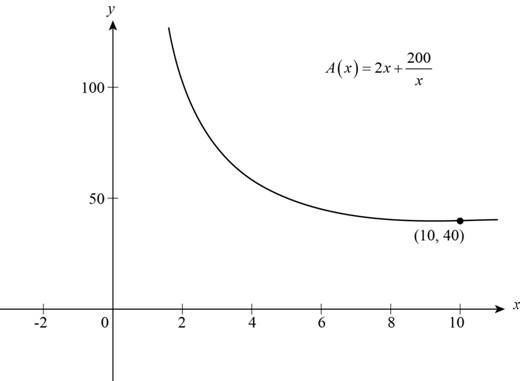# The function that models the length of fencing required to build a rectangular pen whose area is 100 m 2 .### Precalculus: Mathematics for Calcu...

6th Edition
Stewart + 5 others
Publisher: Cengage Learning
ISBN: 9780840068071### Precalculus: Mathematics for Calcu...

6th Edition
Stewart + 5 others
Publisher: Cengage Learning
ISBN: 9780840068071

#### Solutions

Chapter 2, Problem 29P

(a)

To determine

## To find: The function that models the length of fencing required to build a rectangular pen whose area is 100 m2 .

Expert Solution

The function that models the length of fencing is A(x)=2x+200x .

### Explanation of Solution

Let the dimension of the rectangular pen be x and y.

Perimeter (length of fencing) of rectangular pen is,

P=2(x+y)

Area of rectangular fence is product of its dimensions.

Area=xy

Substitute 100 for Area in above equation and get y in terms of x.

100=xy

Divide the above equation by x.

100x=y

Summarize the information in the table as shown below.

 In Words In Algebra Length of fencing P Length of rectangular pen. x Width of the rectangular pen 100x

Use the information and model the function.

P=2(x+y)P(x)=2(x+100x)=2x+200x

Thus, the function that models the length of fencing is A(x)=2x+200x .

(b)

To determine

### To find: The dimension of pen that requires minimum amount of fencing.

Expert Solution

The dimension of fencing for minimum length is 10 m by 10 m.

### Explanation of Solution

The function that models the length of fencing as calculated in part (a) is,

A(x)=2x+200x

Sketch the graph of length function as shown below.Figure (1)

Observe from Figure (1) that function attains a minimum value when x is 10.

Substitute 10 for x in equation (2) and solve for y.

100=10yy=10010y=10

The value of y is 10m .

Thus, the dimension of fencing for minimum length is 10 m by 10 m.

### Have a homework question?

Subscribe to bartleby learn! Ask subject matter experts 30 homework questions each month. Plus, you’ll have access to millions of step-by-step textbook answers!